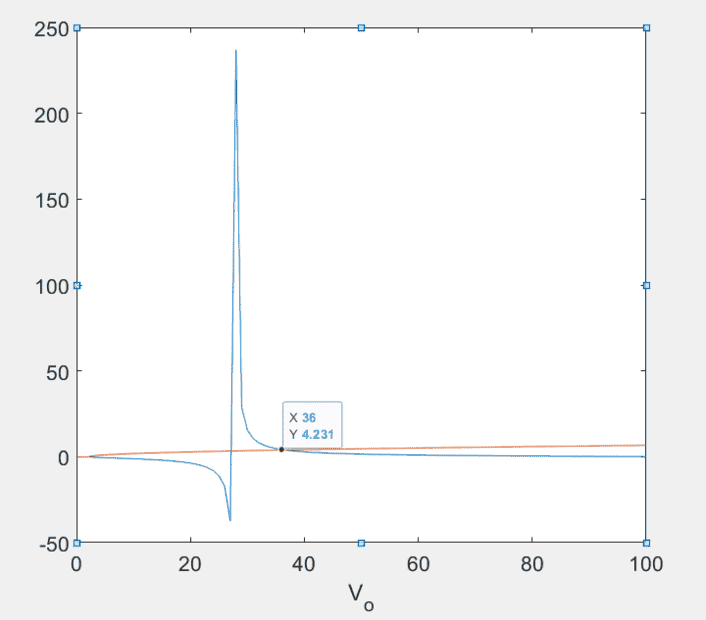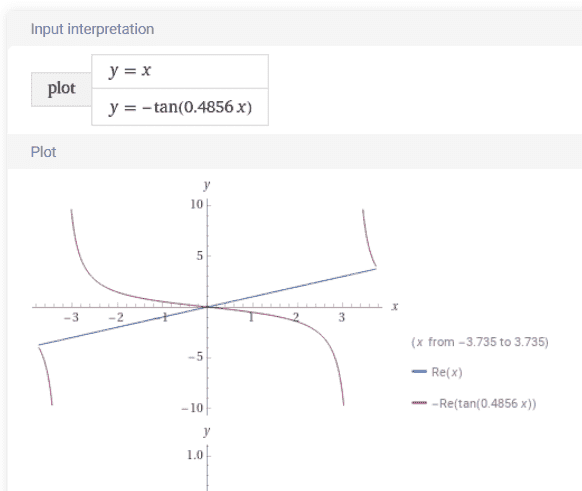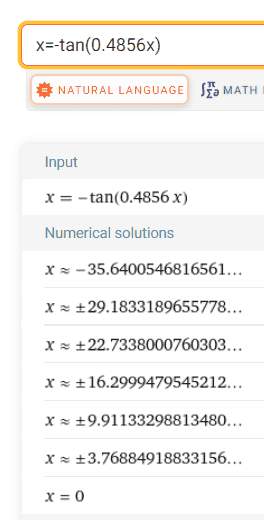# Deuteron Transcendental Equation

James_1978
Homework Statement:
I believe I solved the transcendental equation but the plot does not make sense.
Relevant Equations:
##k_{1} \cot{k_{1}R} = -k_{2}##
##k_{1} = \frac{\sqrt{2m(E+V_{o})}}{\hbar}##
##k_{2} = \frac{\sqrt{-2mE}}{\hbar}##
##x = -\tan{bx}##
##x = \sqrt{\frac{-(V_{o} + E)}{E}}##
Dear Forum,

I am trying to solve a problem (4.6) from the introductory nuclear physics textbook by Krane. The problem is as follows:
Solving the deuteron using the radial equations gives the transcendental function,

##k_{1} \cot{k_{1}R} = -k_{2}##

Were

##k_{1} = \frac{\sqrt{2m(E+V_{o})}}{\hbar}##

And

##k_{2} = \frac{\sqrt{-2mE}}{\hbar}##

That gives the relations between and R. Show that this equation can be written in the form,

##x = -\tan{bx}##

Where

##x = \sqrt{\frac{-(V_{o} + E)}{E}}##

Evaluate the parameter b for R = 2fm. Note that is the reduced mass. Solve the transcendental equation.

When rearranging we get ##b## as.

##b = \frac{\sqrt{-2mE}}{\hbar}*R##

For the reduced mass ##m = \frac{1.67x10^{-27}}{2} kg##
For ##\hbar = 1.054x10^{-34} J-s##
For ##E = -2.22 MeV##

We are suppose to see that when solving the transcendental equation we get ##V_{o} = 36 MeV##. However we must have something wrong because the function does not clearly show how you infer the ##V_{o} = 36 MeV##. Any help is appreciated.

Gold Member
How much value of b you get ? Please let me know it for checking your result.

Last edited:
James_1978
Dear Anuttarasmmyak

Here is what I got for b

##b = \frac{\sqrt{-2*\frac{1.67x10^{-27}}{2}*-2.22*1.602x10^{-13}}}{1.054x10^{-34}}##

Where ##b =2.3155x10^{14} m^{-1}##

Or with R I get ##b*R = 0.4856## I get ##V_{o} = 36##. I think this is correct. Just wanted to make sure.Gold Member
b has no physical dimension.

James_1978
Yes. I saw that. b*R is unit-less. My mistake.

Gold Member
Is MeV translated to MKSA Joule ?

James_1978
Yes. I think you are asking in that I multiplied MeV*1.602x10^-13 to convert MeV to Joules. Is that what you are asking?

Gold Member
And you say R is 2 fm.

James_1978
Yes, I use 2x10-15 m.

Gold Member
Or with R I get
So you say b=0.4856.

James_1978
Yes. That is what I got.

Gold MemberThe first crossing point is approximately x=3.75By numerical solution x=3.7688.. How much V_0 does it give us?

[EDIT] I could read the text in https://www.chegg.com/homework-help...r-physics-3-condition-existence-bou-q51837337 . The author himself investigated V_0=35 Mev. 36 and 35 are not so much different for me.

Last edited:
Staff Emeritus
Homework Helper
So you say b=0.4856.
Yes. That is what I got.
I got a different value for ##b##. I calculated ##b=0.4627##.

James_1978
What did you use for E and mass of proton?

Staff Emeritus